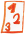Custom math worksheets at your fingertips# Details for problem "Insert relational operator for subtraction terms"

Quickname: 5303

## Summary

The correct relational operator has to be inserted for subtraction terms.

## Example## Description

In a series of problems, the correct relational operator "less than", "greater than", or "equal to" has to be chosen and inserted between to terms. One or both of these terms are either numbers or subtraction terms.

The number of problems can be selected. The number range can be chosen. Both the numbers and the sum will be within that number range.

There are options to choose from on where the subtraction terms will occur:

- random occurrence

- always on one side of the relational operator

- always on both sides of the relational operator

Download free worksheets for this math problem here. The worksheet contains the problems only, the solutions sheet includes the answers. Just click on the respective link.

•Worksheet 1Solution sheet with answers
•Worksheet 2Solution sheet with answers
•Worksheet 3Solution sheet with answers

If you can not see the solution sheets for download, they may be filtered out by an ad blocker that you may have installed. If this is the case, please allow ads for this page and reload the page. The solution sheets will then reappear.

• Do the sample worksheets do not really fit?
• Do you need more math worksheets, with a different level of difficulty?
• Would you like to combine different problems on a worksheet and adjust them to your needs?
• As a teacher, you can put together your own worksheets using the automatically generated math problems provided.
With a free initial credit, you can start creating your own math worksheets in a few minutes.

It does not cost anything to try! Register here, to create custom worksheets now!

## Customization options for this problem

Parameter
Possible values
Number of problems
1, 2, 3, 4, 5, 6, 7, 8, 9, 10
Number range
10, 20, 30, 40, 50, 100, 200, 500, 1000, 10000, 100000
Terms on sides
random, one side, both sides

Remark
Description